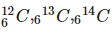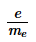Courses

# Structure Of Atom - Practice Test(1)

## 25 Questions MCQ Test Additional Question Bank | Structure Of Atom - Practice Test(1)

Description
This mock test of Structure Of Atom - Practice Test(1) for Class 9 helps you for every Class 9 entrance exam. This contains 25 Multiple Choice Questions for Class 9 Structure Of Atom - Practice Test(1) (mcq) to study with solutions a complete question bank. The solved questions answers in this Structure Of Atom - Practice Test(1) quiz give you a good mix of easy questions and tough questions. Class 9 students definitely take this Structure Of Atom - Practice Test(1) exercise for a better result in the exam. You can find other Structure Of Atom - Practice Test(1) extra questions, long questions & short questions for Class 9 on EduRev as well by searching above.
QUESTION: 1

### According to Dalton’s atomic theory

Solution:

According to Daltons atomic theory atoms are indivisible.

QUESTION: 2

### Which of these is not part of Bohr’s theory for hydrogen atom?

Solution:

Bohr did not talk about the wave nature of electron.

QUESTION: 3

### Experiments on electrical discharge through gases ultimately lead to the discovery of

Solution:

This lead to the discovery of electrons.

QUESTION: 4

The commonly occurring isotopes of carbon atoms are:. They contain:

Solution:

P+N = mass number While P = atomic number.

QUESTION: 5

Given a spectral line of the Balmer series, what happens when the electron in a hydrogen atom undergoes transition from an energy level with n = 4 to an energy level with n = 2?

Solution:

On deexcitation a spectral line will appear in visible regaion because Balmar series lies in visible region of EM spectrum.

QUESTION: 6

Cathode rays or cathode ray particles are

Solution:

Cathode rays have electron particles.

QUESTION: 7

According to the Heisenberg uncertainty principle, in dealing with milligram-sized or heavier objects,

Solution:

For any macroscopic particle there is no uncertainity involved.

QUESTION: 8

Television pictures result due to

Solution:

Television pictures result due to fluorescence of cathode rays on the television screen

QUESTION: 9

How many protons, neutrons and electrons are in  56Fe3+?

Solution:

Fe has atomic number 26 so P= 26 while P+N=56. Also since it has 3 unit of positive charge means E=26-3=23

QUESTION: 10

Bohr’s model of atom explains spectrum of

Solution:

Bohr’s model of atom explains spectra of hydrogen atom or hydrogen like species ( having 1 electron).

QUESTION: 11

J.J. Thomson measured the ratio of electrical charge (e) to the mass of electron (me) by using cathode ray tube and applying electrical and magnetic field perpendicular to each other as well as to the path of electrons. His experiments showed that

Solution:

value ofis constant

QUESTION: 12

Quantum mechanics is a theoretical science that deals with the study of the

Solution:

Quantum mechanics is a theoretical science that deals with the study of the motions of the microscopic objects that have both observable wave like and particle like properties.

QUESTION: 13

The mass of an electron is

Solution:

=The mass of an electron is 10−31 kg

QUESTION: 14

Give the number of electrons in the species, O2 and O2+ .

Solution:

E= atomic number O has atomic number =8 so number of electrons in O2 = 16 while in O2+ there is one unit negative charge so no. of electron =15.

QUESTION: 15

The product of the uncertainties of the exact position and exact momentum (or velocity) of an electron. The product of their uncertainties is always

Solution:

Δx. Δp≧ h/4Л

QUESTION: 16

Charge on the electron was determined by

Solution:

Mullikens experiment determines the charge on electron.

QUESTION: 17

According to quantum theoretical model of an atom each orbital is designated by three quantum numbers labelled as n, l and ml. These are referred to respectively as

Solution:

n= principal Quantum no. l= azimuthal Quantum no. ml = magnetic quantum no

QUESTION: 18

The formula E= hνν is used to calculate

Solution:

E= hνν is result of planks Quantum theory which states that energy of EMR is present in form of Quantum.

QUESTION: 19

Lines in the hydrogen spectrum which appear in the visible region of the electromagnetic Spectrum, then they are called as

Solution:

Balmar series lies in visible region of Electromagnetic spectrum

QUESTION: 20

in the Thomson Model of Atom

Solution:

In the Thomson Model of Atom electrons are embedded in a positively charged pudding or water melon.

QUESTION: 21

According to quantum theoretical model of an atom each orbital is designated by three quantum numbers labelled as n, l and ml. The values these can take are

Solution:

n=1,2,3,4…. l= 0 to n-1 while ml = -l to +l.

QUESTION: 22

Wave number of yellow radiations having wavelength of 5800 A0A0.

Solution:

waveno. = 1/wavelength

QUESTION: 23

An atom of an element contains 29 electrons and 35 neutrons. The number of protons are:

Solution:

In a atom no. of protons = no. of electrons i.e. P=E while this is not true in case of ions.

QUESTION: 24

Using s,p,d and f notation, choose the orbital with n=4, l=3 quantum numbers

Solution:

For l=3 orbital that exist is f.

QUESTION: 25

Rutherford’s α–particle scattering experiment supported the discovery of

Solution:

Ruferford gold foil experiment proved the existence of nucleus.

• TEST1